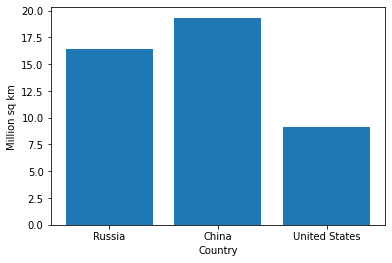# pyplot : Sample code to draw a graph using python like MATLAB.

matplotlib.pyplot is a collection of functions that make matplotlib work like MATLAB. pyplot function can creates plotting area in a figure, plots some lines in a plotting area, decorates the plot with labels, etc.

Sample code

``````import matplotlib.pyplot as pyplot
labels = ('Russia', 'China', 'United States')
index = (1, 2, 3) # x axis
sizes = [16.38, 19.330, 9.16 ]
pyplot.bar(index, sizes, tick_label=labels)
pyplot.ylabel('Million sq km')
pyplot.xlabel('Country')
``````

ResultAuthor: user